# 【Javascript】Sizzle引擎--原理与实践（二）

2012-02-16

## 主要流程与正则

### 表达式分块

``````var chunker = /((?:\((?:\([^()]+\)|[^()]+)+\)|\[(?:\[[^[\]]*\]|['"][^'"]*['"]|[^[\]'"]+)+\]|\\.|[^ >+~,(\[\\]+)+|[>+~])(\s*,\s*)?((?:.|\r|\n)*)/g;
``````

1、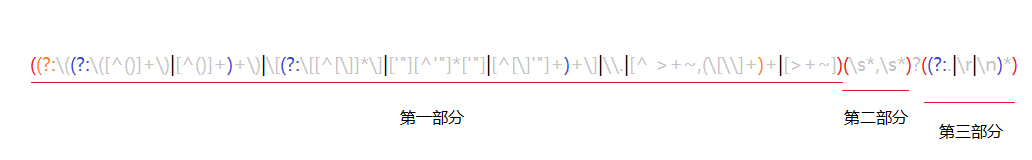2、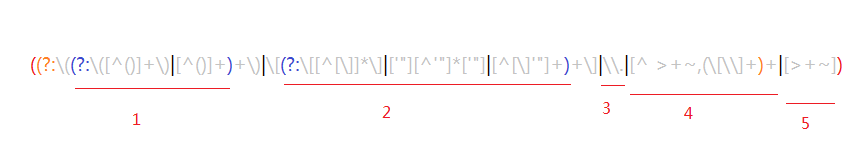3、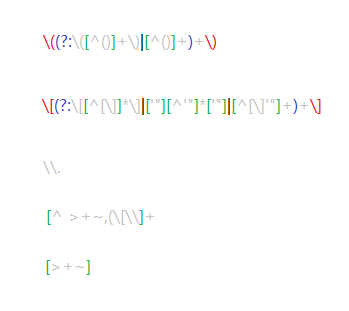4、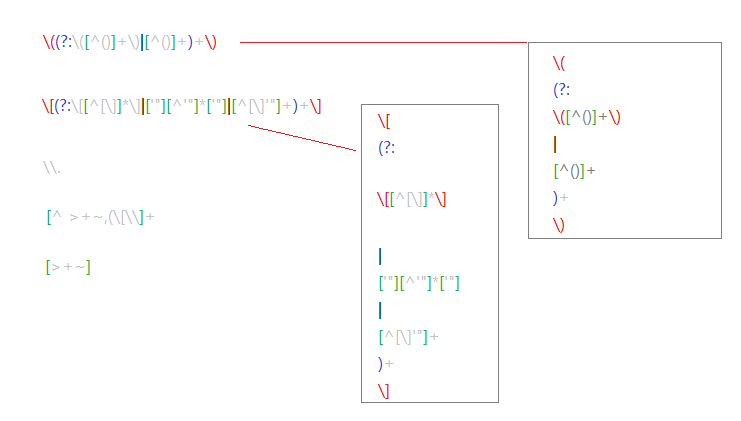``````'div#test + p > a.tab'    --> ['div#test','+','p','>','a.tab']
``````

### 从表达式提取出相应的类型:

``````ID选择器
CLASS选择器
TAG选择器
ATTR属性选择器
CHILD子元素选择器
PSEUDO伪类选择器
POS位置选择器
``````

``````ID    : /#((?:[\w\u00c0-\uFFFF\-]|\\.)+)/,
CLASS : /\.((?:[\w\u00c0-\uFFFF\-]|\\.)+)/,
NAME  : /\[name=['"]*((?:[\w\u00c0-\uFFFF\-]|\\.)+)['"]*\]/,
ATTR  : /\[\s*((?:[\w\u00c0-\uFFFF\-]|\\.)+)\s*(?:(\S?=)\s*(?:(['"])(.*?)\3|(#?(?:[\w\u00c0-\uFFFF\-]|\\.)*)|)|)\s*\]/,
TAG   : /^((?:[\w\u00c0-\uFFFF\*\-]|\\.)+)/,
CHILD : /:(only|nth|last|first)-child(?:\(\s*(even|odd|(?:[+\-]?\d+|(?:[+\-]?\d*)?n\s*(?:[+\-]\s*\d+)?))\s*\))?/,
POS   : /:(nth|eq|gt|lt|first|last|even|odd)(?:\((\d*)\))?(?=[^\-]|\$)/,
PSEUDO: /:((?:[\w\u00c0-\uFFFF\-]|\\.)+)(?:\((['"]?)((?:\([^\)]+\)|[^\(\)]*)+)\2\))?/
``````

ID: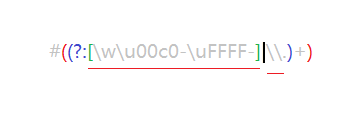CLASS: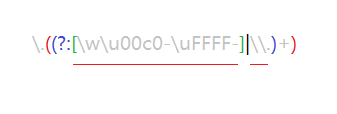NAME: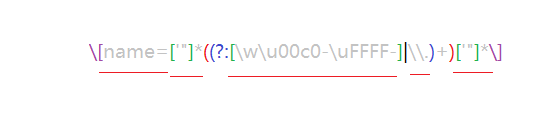TAG: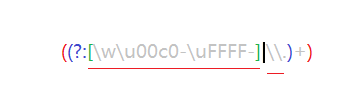ATTR:POS: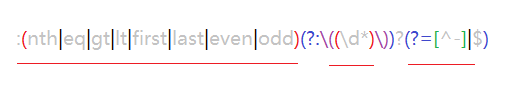PSEUDO: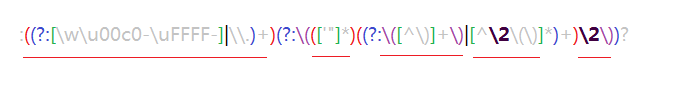``````？ 非贪婪量词
\3 匹配分
?= 正向预查
``````

``````POS   :first :nth() :last :gt :lt :even :odd
``````

``````Expr = {
match:{
//ID:....
}
}
``````

``````/#((?:[\w\u00c0-\uFFFF\-]|\\.)+))/变成/#((?:[\w\u00c0-\uFFFF\-]|\\.)+)(?![^\[]*\])(?![^\(]*\)/
``````

``````/#((?:[\w\u00c0-\uFFFF\-]|\\.)+)(?![^\[]*\])(?![^\(]*\)/

/(^(?:.|\r|\n)*?)#((?:[\w\u00c0-\uFFFF\-]|\\.)+)(?![^\[]*\])(?![^\(]*\)/

/:((?:[\w\u00c0-\uFFFF\-]|\\.)+)(?:\((['"]?)((?:\([^\)]+\)|[^\(\)]*)+)\2\))?/

/(^(?:.|\r|\n)*?):((?:[\w\u00c0-\uFFFF\-]|\\.)+)(?:\((['"]?)((?:\([^\)]+\)|[^\(\)]*)+)\3\))?(?![^\[]*\])(?![^\(]*\)/
``````

``````    var fescape = function(all, num){
return "\\" + (num - 0 + 1);
};

for ( var type in Expr.match ) {
Expr.match[ type ] = new RegExp( Expr.match[ type ].source + (/(?![^\[]*\])(?![^\(]*\))/.source) );
Expr.leftMatch[ type ] = new RegExp( /(^(?:.|\r|\n)*?)/.source + Expr.match[ type ].source.replace(/\\(\d+)/g, fescape) );
}
``````

Expr.leftMatch 中保存的是处理过后的正则部分，这么做的另一个好处就是避免每次匹配都去创建一个新的RegExp对象

### 回到主流程

``````var Sizzle = function( selector, context, results, seed ){}
``````

Sizzle有四个参数：

selector ：选择表达式 　　context ：上下文 　　results ：结果集 　　seed ：候选集

``````Sizzle('div',#test,[#a,#b],[#c,#d,#e])
``````

#### 代码示例

``````    var Sizzle = function( selector, context, results, seed ){
var soFar = selector,
extra    ,//extra用来保存并联选择的其他部分，一次只处理一个表达式
parts = [],
m;
do {
chunker.exec( "" );     //这一步主要是将chunker的lastIndex重置，当然直接设置chunker.lastIndex效果也一样
m = chunker.exec( soFar );
if ( m ) {
soFar = m;
parts.push( m );
if ( m ) {       //如果存在并联选择器，就中断，保存其他的选择器部分。
extra = m;
break;
}
}
} while ( m );
}
``````

parts结果就是 [‘div#test’,’+’,’p’,’>’,’a.tab’]

``````    if ( parts.length > 1 && origPOS.exec( selector ) ) {
//自左向右，判断标准就是存在关系选择符同时有位置选择符，因为如果只是类似div#test的选择表达式，就不存在顺序的问题。
}else{
//其他，自右向左
}
``````

【说明：origPOS保存的是Expr.match.POS，源码901行】

``````ret = seed ?{ expr: parts.pop(), set: makeArray(seed) } :Sizzle.find( parts.pop(), context);//Sizzle.find负责查找
set = ret.expr ? Sizzle.filter( ret.expr, ret.set ) : ret.set;//Sizzle.filter负责过滤
``````

``````    while ( parts.length ) {
Expr.relative[ cur ]( checkSet, context, contextXML );//context代表上下文，并非源码中的参数
}
``````

#### 实例说明：

``````['div#test','+','p','>','a.tab']
``````

``````第一步、没有候选集seed，第一项'div#test'中含有id信息，最后一项'a.tab'中不含有id信息，因此重设content=Sizzle.find('div#test',document)

C = [#a,#b,false,false],然后获取紧挨第一步中content的元素，获得集合D = [#a,false,false,false]

``````

## 讨论

#### 实例说明

``````<body>
<div id="test_a">
<p class="tab" id="a1">a1</p>
<p class="tab" id="a1">a2</p>
<p class="tab" id="a1">a3</p>
</div>
<div id="test_b">
<p class="tab" id="b1">b1</p>
<p class="tab" id="b1">b2</p>
<p class="tab" id="b1">b3</p>
</div>
</body>
``````

``````'div#test_a ~ div'
``````